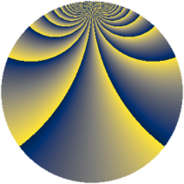# Properties

 Label 2280.2.mLevel $2280$ Weight $2$ Character orbit 2280.m Rep. character $\chi_{2280}(1709,\cdot)$ Character field $\Q$ Dimension $472$ Sturm bound $960$

# Related objects

## Defining parameters

 Level: $$N$$ $$=$$ $$2280 = 2^{3} \cdot 3 \cdot 5 \cdot 19$$ Weight: $$k$$ $$=$$ $$2$$ Character orbit: $$[\chi]$$ $$=$$ 2280.m (of order $$2$$ and degree $$1$$) Character conductor: $$\operatorname{cond}(\chi)$$ $$=$$ $$2280$$ Character field: $$\Q$$ Sturm bound: $$960$$

## Dimensions

The following table gives the dimensions of various subspaces of $$M_{2}(2280, [\chi])$$.

Total New Old
Modular forms 488 488 0
Cusp forms 472 472 0
Eisenstein series 16 16 0

## Trace form

 $$472q - 4q^{4} - 12q^{6} - 8q^{9} + O(q^{10})$$ $$472q - 4q^{4} - 12q^{6} - 8q^{9} - 12q^{16} - 52q^{24} - 8q^{25} - 16q^{30} - 28q^{36} + 16q^{39} - 440q^{49} - 20q^{54} - 48q^{55} - 52q^{64} - 16q^{66} + 36q^{76} - 8q^{81} - 60q^{96} + O(q^{100})$$

## Decomposition of $$S_{2}^{\mathrm{new}}(2280, [\chi])$$ into newform subspaces

The newforms in this space have not yet been added to the LMFDB.